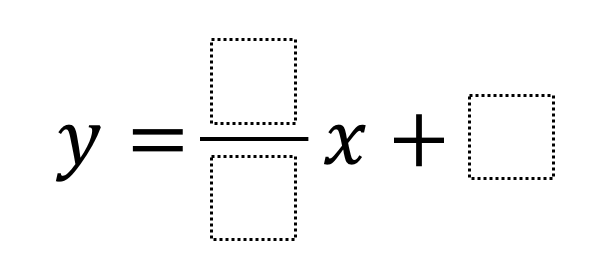Home > Grade 8 > Functions > Write a Linear Function

# Write a Linear Function

Directions: Using the whole numbers 1 through 8 [You will use each number only once, except for one number that will be used twice in the same coordinate point. i.e.(1,1), (2,2), (3,3), (4,4), (5,5), (6,6), (7,7) or (8,8)], find three coordinate points that lie on the same line. Write the equation of the line represented by the three points and have the following requirements:
a.) It has a positive slope
b.) The slope is less than one.### Hint

What does it mean for the slope to be positive and less than one?
How does this affect the coordinates, specifically being restricted by the numbers you can use?